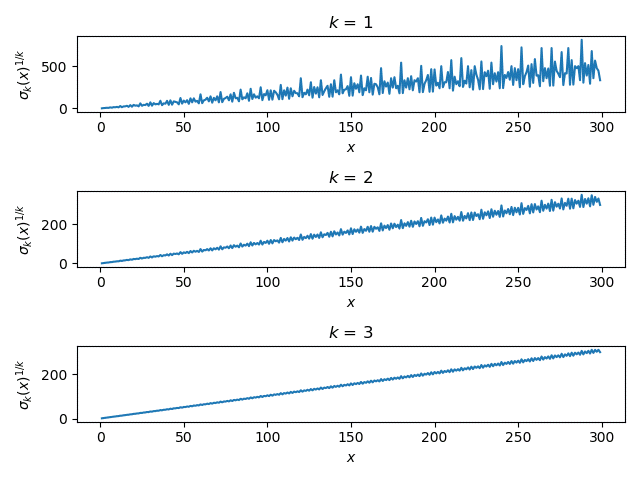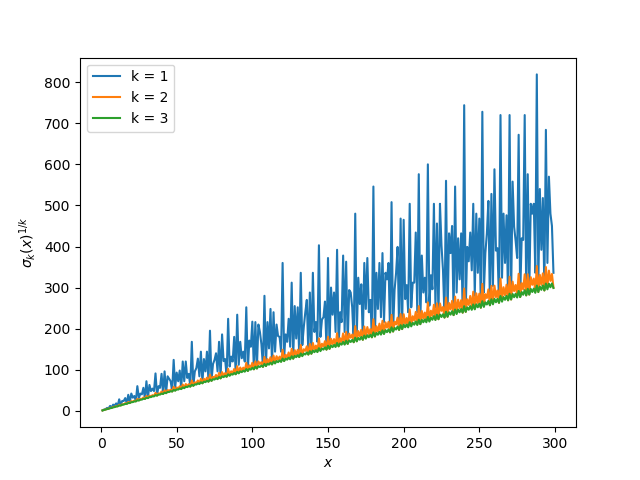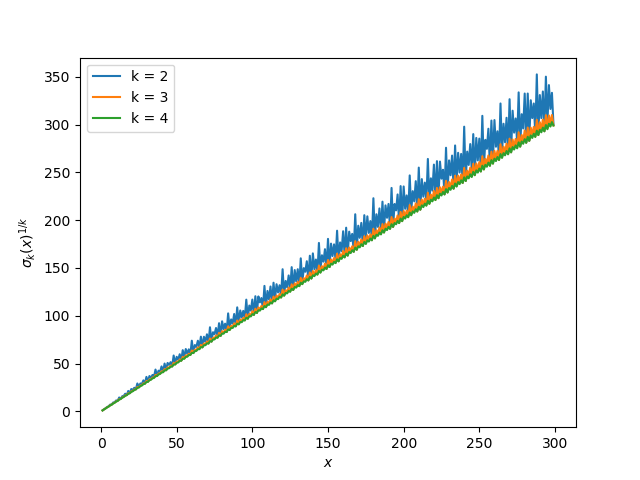# Sum of divisor powers

The function σk takes an integer n and returns the sum of the kth powers of divisors of n. For example, the divisors of 14 are 1, 2, 4, 7, and 14. If we set k = 3 we get

σ3(n) = 1³ + 2³ + 4³ + 7³ + 14³ = 3096.

A couple special cases may use different notation.

• σ1(n) is the sum of the divisors of n and the function is usually written σ(n) with no subscript.

In Python you can compute σk(n) using `divisor_sigma` from SymPy. You can get a list of the divisors of n using the function `divisors`, so the bit of code below illustrates that `divisor_sigma` computes what it’s supposed to compute.

```    n, k = 365, 4
a = divisor_sigma(n, k)
b = sum(d**k for d in divisors(n))
assert(a == b)
```

The Wikipedia article on σk gives graphs for k = 1, 2, and 3 and these graphs imply that σk gets smoother as k increases. Here is a similar graph to those in the article.The plots definitely get smoother as k increases, but the plots are not on the same vertical scale. In order to make the plots more comparable, let’s look at the kth root of σk(n). This amounts to taking the Lebesgue k norm of the divisors of n.Now that the curves are on a more similar scale, let’s plot them all on a single plot rather than in three subplots.If we leave out k = 1 and add k = 4, we get a similar plot.The plot for k = 2 that looked smooth compared to k = 1 now looks rough compared to k = 3 and 4.

## 2 thoughts on “Sum of divisor powers”

1. When you take the kth root, for large k you’re close to looking at the largest divisor of n, which is just n. That may help explain the smoothing.

2. David v. Rudisill

Didn’t Ramanujan do work in this area? This looks like it’s related to the tau function.